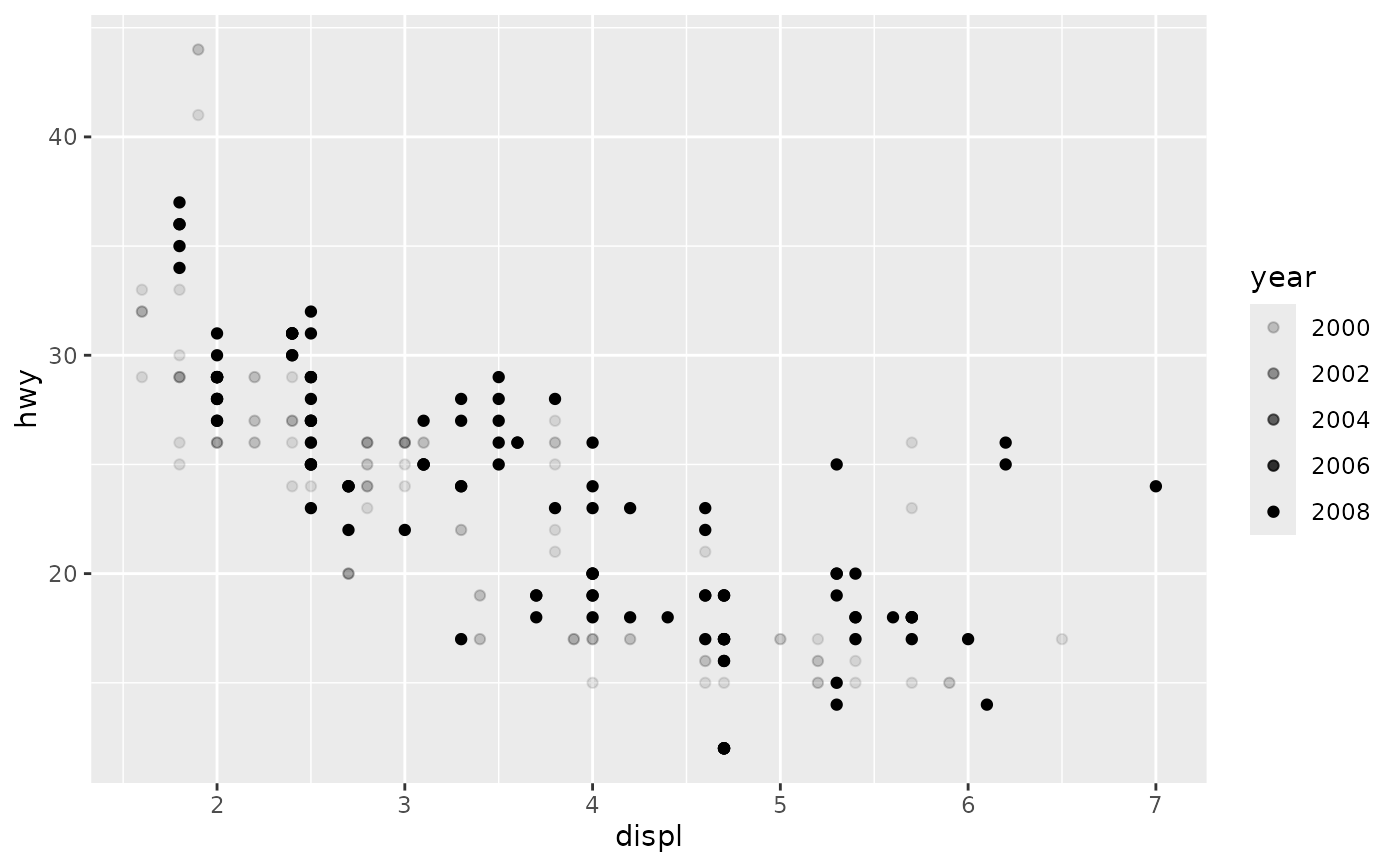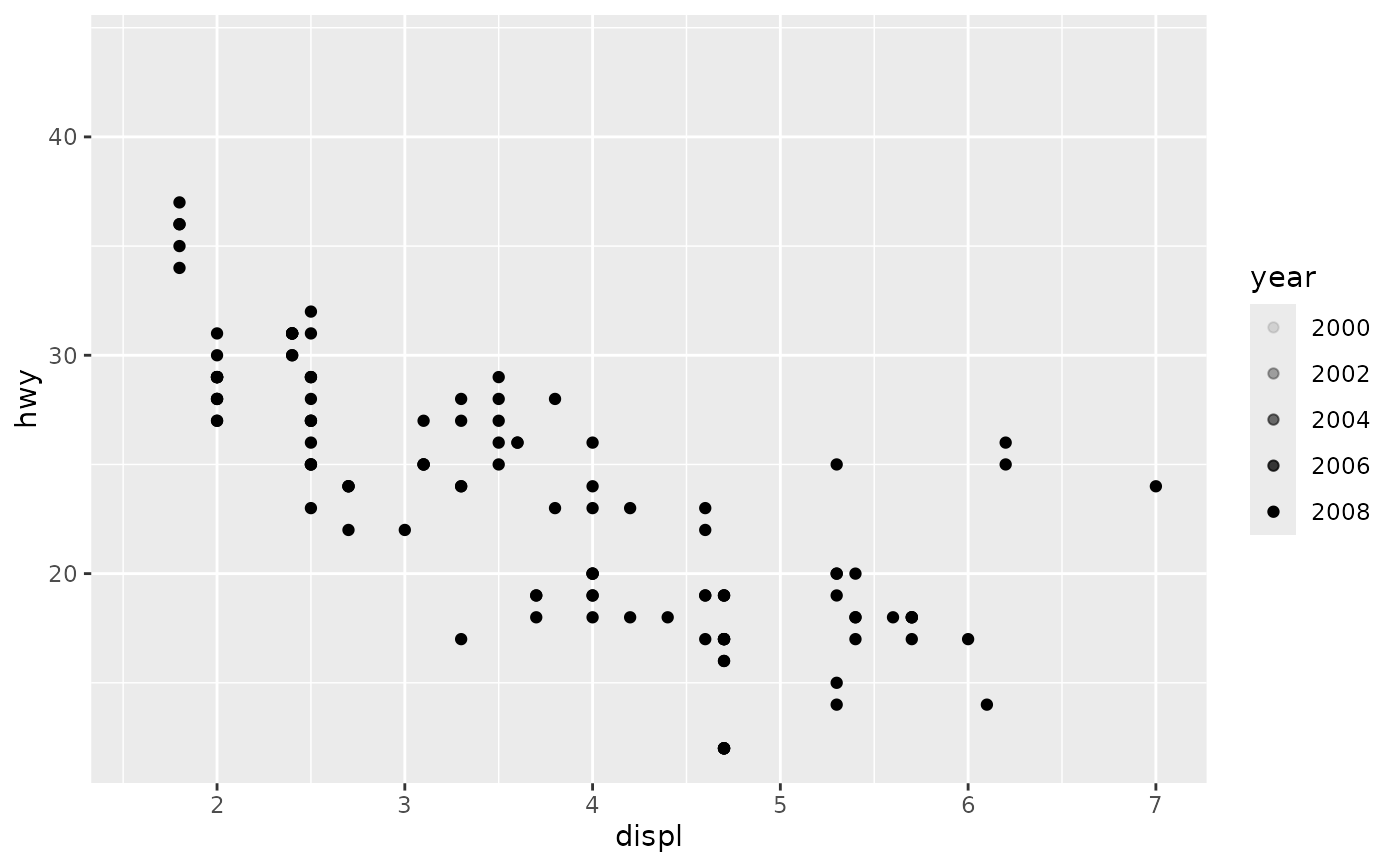Alpha-transparency scales are not tremendously useful, but can be a convenient way to visually down-weight less important observations. scale_alpha() is an alias for scale_alpha_continuous() since that is the most common use of alpha, and it saves a bit of typing.

## Usage

scale_alpha(..., range = c(0.1, 1))

scale_alpha_continuous(..., range = c(0.1, 1))

scale_alpha_binned(..., range = c(0.1, 1))

scale_alpha_discrete(...)

scale_alpha_ordinal(..., range = c(0.1, 1))

## Arguments

...

Other arguments passed on to continuous_scale(), binned_scale(), or discrete_scale() as appropriate, to control name, limits, breaks, labels and so forth.

range

Output range of alpha values. Must lie between 0 and 1.

Other colour scales: scale_colour_brewer(), scale_colour_continuous(), scale_colour_gradient(), scale_colour_grey(), scale_colour_hue(), scale_colour_steps(), scale_colour_viridis_d()

## Examples

p <- ggplot(mpg, aes(displ, hwy)) +
geom_point(aes(alpha = year))

pp + scale_alpha("cylinders")p + scale_alpha(range = c(0.4, 0.8))